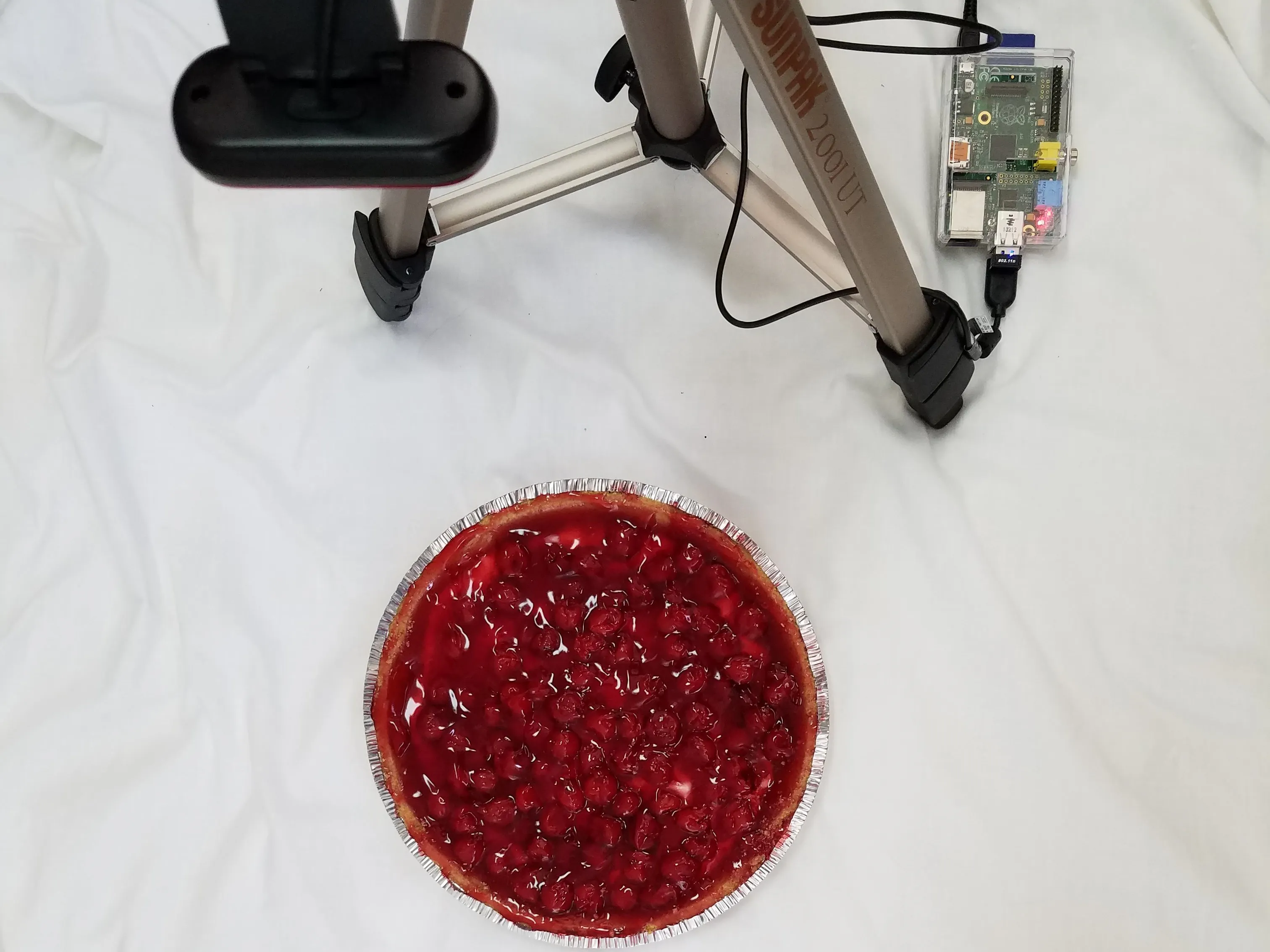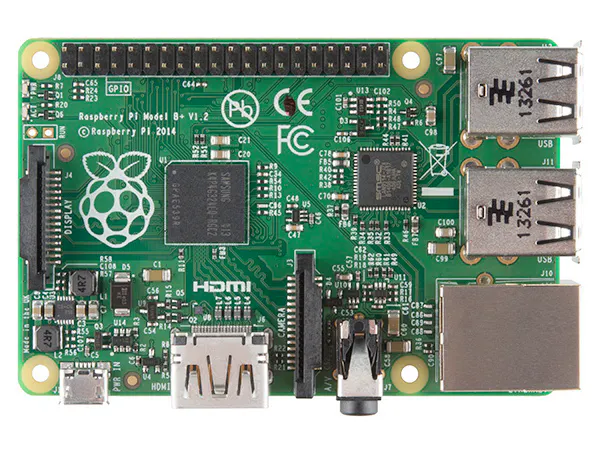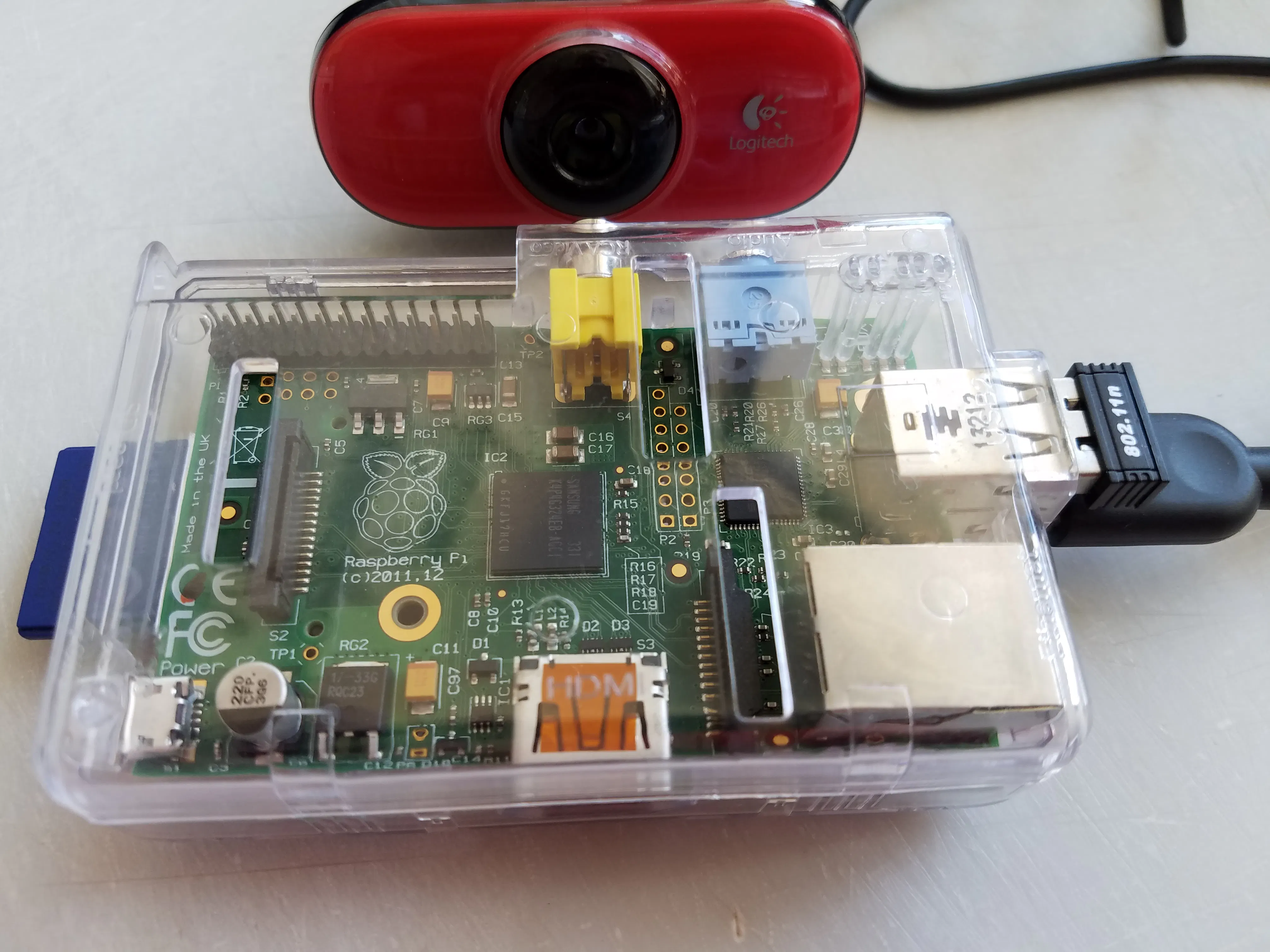Calculate Pi with a Raspberry Pi and a Circular Cherry Pie

I celebrated Pi Day by making a cherry pie, and used a Raspberry Pi with computer vision to take an image of it, and calculate Pi=area/R**2.

Intermediate8 hours1,337Things used in this project

Hardware componentsRaspberry Pi 1 Model B+
×1

Software apps and online services

 SimpleCV in Python

Schematics

Raspberry Pi and a USB Camera

I used the SimpleCV library to drive the cameraCode

Pi calculator using a camera to take images of a pie.

Python
Need SimpleCV installed on Python 2.7.3
from time import sleep
from SimpleCV import *

cam = Camera()
files = []
j=1

#take 5 photos, 2 seconds apart, and same them as jpegs

while j <= 5:
pie_image = cam.getImage()
filename="pie_"+str(j)+".jpg"
pie_image.save(filename)
files.append(filename)
j=j+1
sleep(2)

#find the pie blob in each photo and calculate pi

k=1
for i in files:
img = Image(i)
segmented = img.hueDistance(Color.BLACK)
pies = img.invert().findBlobs(minsize=20000)

#Now we print the measurements back on the picture
for pie in pies:
print(round(pi,5))
text = "Pi:" + str(round(pi,4))
img.drawText(text, pie.x, pie.y, fontsize=14)

#img.show()
img.save("pie_pi_w_picalcs_"+str(k)+".jpg")
k=k+1
time.sleep(.1)

Credits

Dave Vaughan

1 project • 1 follower